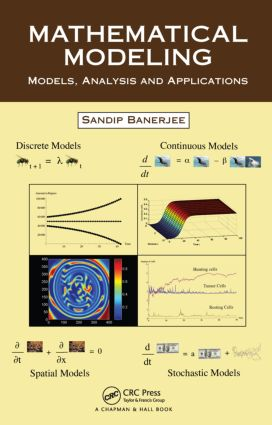# Mathematical Modeling

## Models, Analysis and Applications, 1st Edition

Chapman and Hall/CRC

276 pages | 77 B/W Illus.

Hardback: 9781439854518
pub: 2014-02-07
SAVE ~\$22.00
\$110.00
\$88.00
x
eBook (VitalSource) : 9780429068676
pub: 2014-02-07
from \$55.00

FREE Standard Shipping!

### Description

Almost every year, a new book on mathematical modeling is published, so, why another? The answer springs directly from the fact that it is very rare to find a book that covers modeling with all types of differential equations in one volume. Until now. Mathematical Modeling: Models, Analysis and Applications covers modeling with all kinds of differential equations, namely ordinary, partial, delay, and stochastic. The book also contains a chapter on discrete modeling, consisting of differential equations, making it a complete textbook on this important skill needed for the study of science, engineering, and social sciences.

More than just a textbook, this how-to guide presents tools for mathematical modeling and analysis. It offers a wide-ranging overview of mathematical ideas and techniques that provide a number of effective approaches to problem solving. Topics covered include spatial, delayed, and stochastic modeling. The text provides real-life examples of discrete and continuous mathematical modeling scenarios. MATLAB® and Mathematica® are incorporated throughout the text. The examples and exercises in each chapter can be used as problems in a project.

Since mathematical modeling involves a diverse range of skills and tools, the author focuses on techniques that will be of particular interest to engineers, scientists, and others who use models of discrete and continuous systems. He gives students a foundation for understanding and using the mathematics that is the basis of computers, and therefore a foundation for success in engineering and science streams.

### Reviews

"…the book is rich with examples. There are examples from ecology, physics, chemistry, economy, medicine, sociology, epidemiology, and more, including specific examples that could be of interest in computer simulation … there are many solved problems and exercises … Both features makes the book a good selection for drawing out examples, problems, and exercises to show what differential equations have to offer to the aspiring modeler …"

Computing Reviews, October 2014

"…the reader may find quite a few interesting examples illustrating several important methods used in applied mathematics. … it may be well used as a valuable source of interesting examples as well as complementary reading in a number of courses."

—Svitlana P. Rogovchenko, Zentralblatt MATH 1298

What Is Mathematical Modeling?

History of Mathematical Modeling

Latest Development in Mathematical Modeling

Various Functional Forms in Mathematical Modeling

Merits and Demerits in Mathematical Modeling

Mathematically Modeling Discrete Process

Difference Equations

Introduction to Discrete Models

One Dimensional Density Independent Models

One Dimensional Density Dependent Models

Stability Analysis

Nonlinear Two-Dimensional Models: Stability and Bifurcation

Continuous Models Using Ordinary Differential Equations

Introduction to Continuous Models

Formation of Various Continuous Models

Stability and Linearization

Phase Plane Diagrams of Linear Systems

Stability Characteristics

Null Cline Approach

Poincare Bendixson Theory

Bifurcation and Limit Cycle

Spatial Models Using Partial Differential Equations

Diffusion Equations: Observations and Consequences

Dispersal Models Using Diffusion

Effect of Density Dependent Dispersal on Population Dynamics

Models for Age Dependent Dispersal

Steady State Solutions and Traveling Waves

Non Linear Stability Analysis for Diffusion Reaction System

Bifurcation Analysis and Limit Cycles

Numerical Solutions of Differential Equations with Diffusion

Pattern Developing Instability in Diffusion Reaction Systems

Effect of Cross Diffusion

Modeling with Delay Differential Equations

An Introduction to Delay Differential Equations

Various Mathematical Models with Time Delay

Delay Induced Model for Tumor Immune Interaction (Single Discrete Delay)

Stage Structure Predator Prey Models with Multiple Delays and Its Global Stability

Models with Distributed Delays

Modeling with Stochastic Differential Equations

Stochastic Process and Stochastic Differential Equations: a Preview

Effect of Additive Noise on a Logistic Equation

Stochastic Stability: Asymptotically Mean Square Stable

Mean Square Stability of a Stochastic Model with Time Delays

Numerical Simulation of Stochastic Differential Equations

Stochastic Metapopulation Models

Examples and Exercises appear at the end of each chapter.